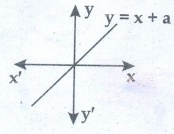Home | | Physics 11th std | Short Questions and Answer

## Chapter: 11th Physics : UNIT 11 : Waves

Physics : Waves : Book Back Important Questions, Answers, Solutions : Short Questions and Answer

Waves (Physics)

1. What is meant by waves?.

The disturbance which carries energy and momentum from one point in space to another point in space without the transfer of the medium is known as a wave.

2. Write down the types of waves.

a) Mechanical wave - Waves which require a medium for propagation are known as mechanical waves.

Examples: sound waves, ripples formed on the surface of water, etc.

b) Non mechanical wave - Waves which do not require any medium for propagation are known as non- mechanical waves.

Example: light

Further, waves can be classified into two types a. Transverse waves b. Longitudinal waves

3. What are transverse waves?. Give one example.

● The constituents of the medium oscillate or vibrate about their mean positions in a direction perpendicular to the direction of propagation of waves.

● Example: light (electromagnetic waves)

4. What are longitudinal waves?. Give one example.

● The constituent of the medium oscillate or vibrate about their mean positions in a direction parallel to the direction of propagation of waves.

● Example: Sound waves travelling in air.

5. Define wavelength.

● For transverse waves, the distance between two neighbouring crests or troughs is known as the wavelength.

● For longitudinal waves, the distance between two neighbouring compressions or rarefactions is known as the wavelength.

● The SI unit of wavelength is meter.

6. Write down the relation between frequency, wavelength and velocity of a wave.

● Dimension of wavelength is, [λ] = L;

Frequency f = 1 / Time Period

which implies that the dimension of frequency is, | f | = 1 / [T] = [T−1]

=> [λ f] = [λ] [ f ] = [LT−1] = [Velocity]

Therefore Velocity, λ f = v

● Where v is known as the wave velocity or phase velocity. This is the velocity with which the wave propagates.

● Wave velocity is the distance travelled by a wave in one second.

7. What is meant by interference of waves?.

Interference is a phenomenon in which two waves superimpose to form a resultant wave of greater, lower or the same amplitude.

8. Explain the beat phenomenon.

● When two or more waves superimpose each other with slightly different frequencies, then a sound of periodically varying amplitude at a point is observed.

● This phenomenon is known as beats. The number of amplitude maxima per second is called beat frequency. If we have two sources, then their difference in frequency gives the beat frequency.

● Number of beats per second n = | f 1 f 2| Per second.

9. Define intensity of sound and loudness of sound.

● The intensity of sound is defined as "the sound power transmitted per unit area taken normal to the propagation of the sound wave".

● The loudness of sound is defined as "the degree of sensation of sound produced in the ear or the perception of sound by the listener".

10. Explain Doppler Effect.

When the source and the observer are in relative motion with respect to each other and to the medium in which sound propagates, the frequency of the sound wave observed is different from the frequency of the source. This phenomenon is called Doppler Effect.

11. Explain red shift and blue shift in Doppler Effect.

● The spectral lines of the star are found to shift towards red end of the spectrum (called as red shift) then the star is receding away from the Earth.

● The spectral lines of the star are found to shift towards the blue end of the spectrum (called as blue shift) then the star is approaching Earth.

12. What is meant by end correction in resonance air column apparatus?

The antinodes are not exactly formed at the open end, we have to include a correction, called end correction.

L1 + e = λ / 4  and L2 + e = 3λ / 4

13. Sketch the function y = x + a. Explain your sketch.i) A combination of constant and direct

ii) A fixed amount is added at regular intervals

iii) y = x + a, a suitable conclusion statement would be that,

1) Y is linear with x

2) Y varies linearly with x

3) Y is a linear function of x, y is the intercept

14. Write down the factors affecting velocity of sound in gases.

Pressure, Temperature, Density, Humidity and wind

15. What is meant by an echo?. Explain.

● An echo is a repetition of sound produced by the reflection of sound waves from a wall, mountain or other obstructing surfaces. The speed of sound in air at 20°C is 344 m s−1

● If we shout at a wall which is at 344 m away, then the sound will take 1 second to reach the wall.

● After reflection, the sound will take one more second to reach us. Therefore, we hear the echo after two seconds.

● Scientists have estimated that we can hear two sounds properly if the time gap or time interval between each sound is (1/10)th of a second (persistence of hearing) i.e., 0.1 s. Then,

Velocity = Distance travelled / Time taken ; = 2d / t

2d = 344 × 0.1 = 34.1m; d = 17.2 m

● The minimum distance from a sound reflecting wall to hear an echo at 20°C is 17.2 meter.

Tags : Waves | Physics , 11th Physics : UNIT 11 : Waves
Study Material, Lecturing Notes, Assignment, Reference, Wiki description explanation, brief detail
11th Physics : UNIT 11 : Waves : Short Questions and Answer | Waves | Physics